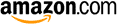Erlang C blending calculatorScenarios INPUT INPUT Forecast per  minutequarterhalf hour INPUT INPUT Average handling time secondsminutes INPUT INPUT Number of agents (integer required) INPUT OUTPUT Threshold (integer required) OUTPUT OUTPUT Productivity % OUTPUT OUTPUT Outbound calls per  minutequarterhalf hour OUTPUT OUTPUT Average speed of answer secondsminutes OUTPUT INPUT Service level % waits less than secondsminutes

Example from "Call Center Optimization" (page 107):
- FC = 5 per minute, AHT = 5 minutes, number of agents = 33, threshold = 5

These calculators are based on CCmath engines.
They can be implemented in any software tool and also be called from within Excel.